# Using the Math Question Generator

## Introduction

The Learnosity math question generator is a tool for content authors that enables easy creation of mathematics questions that use dynamic content.

Math question generator automates the repetitive aspects of mathematics question authoring, by taking a range of values and then creating a data table containing many variations of the question (and relevant solutions), with validation automatically set up.

Math question generator supports all math currently supported in Learnosity and leverages the same scoring methods as other math question types. However, the math question generator applies the scoring methods for you, depending on the type of correct answer you select.

## Availability

Math question generator is available in Learnosity version 2020.1.LTS onwards.

## Usage

To create a math question generator question, add a new item and select the math question generator tile from the math section in the tile view.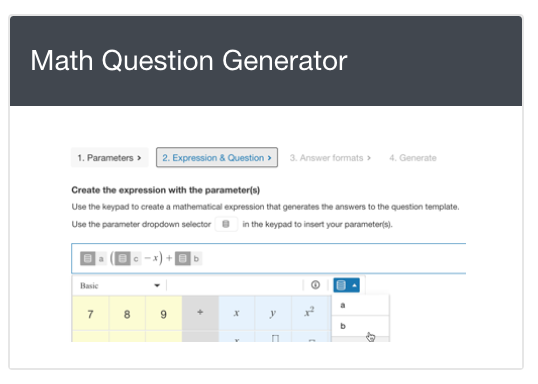Screenshot 1: Math question generator

NOTE: a math question generator question cannot be added to an item with an existingdata table. Also note, you cannot add two math question generator questions in the same item.

Any change to the data table is required to be made within the math question generator wizard and then regenerated.

The math question generator authoring interface contains the dynamic math generator, and settings for the following:

• Settings for layout, including final response box size and associated ARIA label,
• Settings for text blocks,
• General metadata and the math question type, and

When using math question generator on an item for the first time, launch the math question generator wizard by selecting the "Create expression" button.

The dynamic math generator uses a wizard, or step-by-step progression through four screens, designed to step content authors through the creation of algorithmic math content. The four steps are as follows:

1. Create parameters and set their values,
2. Create the expression with the parameter(s),
3. Select the correct answer format(s), and
4. Generate the questions.

## 1. Create parameters and set their values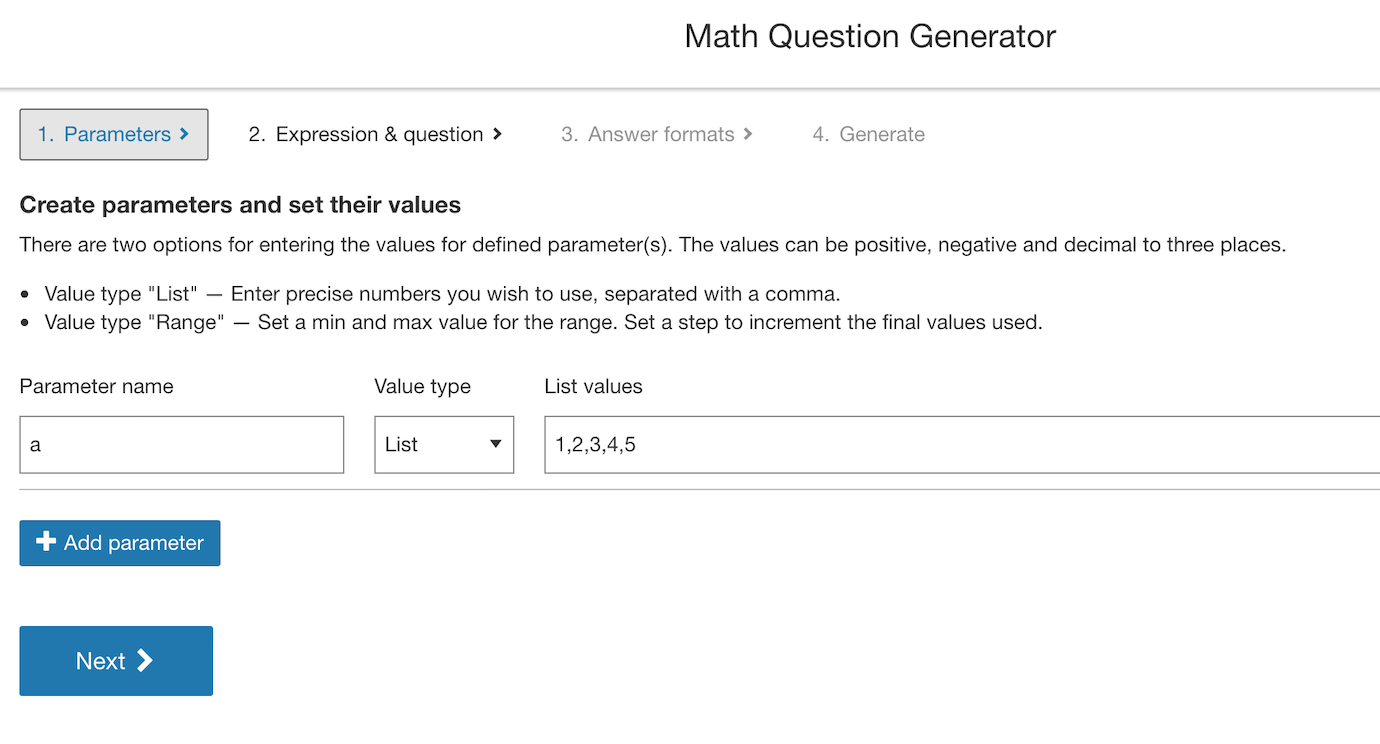Screenshot 2: Creating parameters in math question generator.

In this section, define the parameters that are required for the question. A parameter is any element of an expression that will receive dynamically generated values. Parameters can be added to an expression and also part of the question stem text.

Parameters can only be alpha characters and are used for content creation purposes. They will be exchanged for real values when the item is loaded for the end-user.

Once the parameter name has been defined, value settings are applied for the parameters.

There are two options for setting parameter values, list, and range. Each option will accept positive, negative, and decimal values to three decimal places.

List allows a string of specific, comma-separated values to be defined where there is a need to curate the possible variants.

Range allows setting a minimum and maximum for the range of values to be drawn from. This range can be incremented with the step value. The increment will start at the minimum value and finish at the nearest value to the maximum. The step will not accept negative values, however does support decimals.

Use the add parameter to add a new parameter, parameters can be removed by selecting  'Delete' on the relevant parameter row.

NOTE: There is always a pre-populated parameter, as math question generator requires at least one to operate.

Removing a parameter will remove the column and its associated data in the data table, and it will no longer be available under the 'Data' button. However, if the parameter has already been added to the Composed question field, the parameter instance in the text will not be removed (it must be manually removed).

Once all parameters and values are set, navigate by clicking 'next' or via the breadcrumb link at the top of the generator wizard.

## 2. Create the expression with the parameter(s)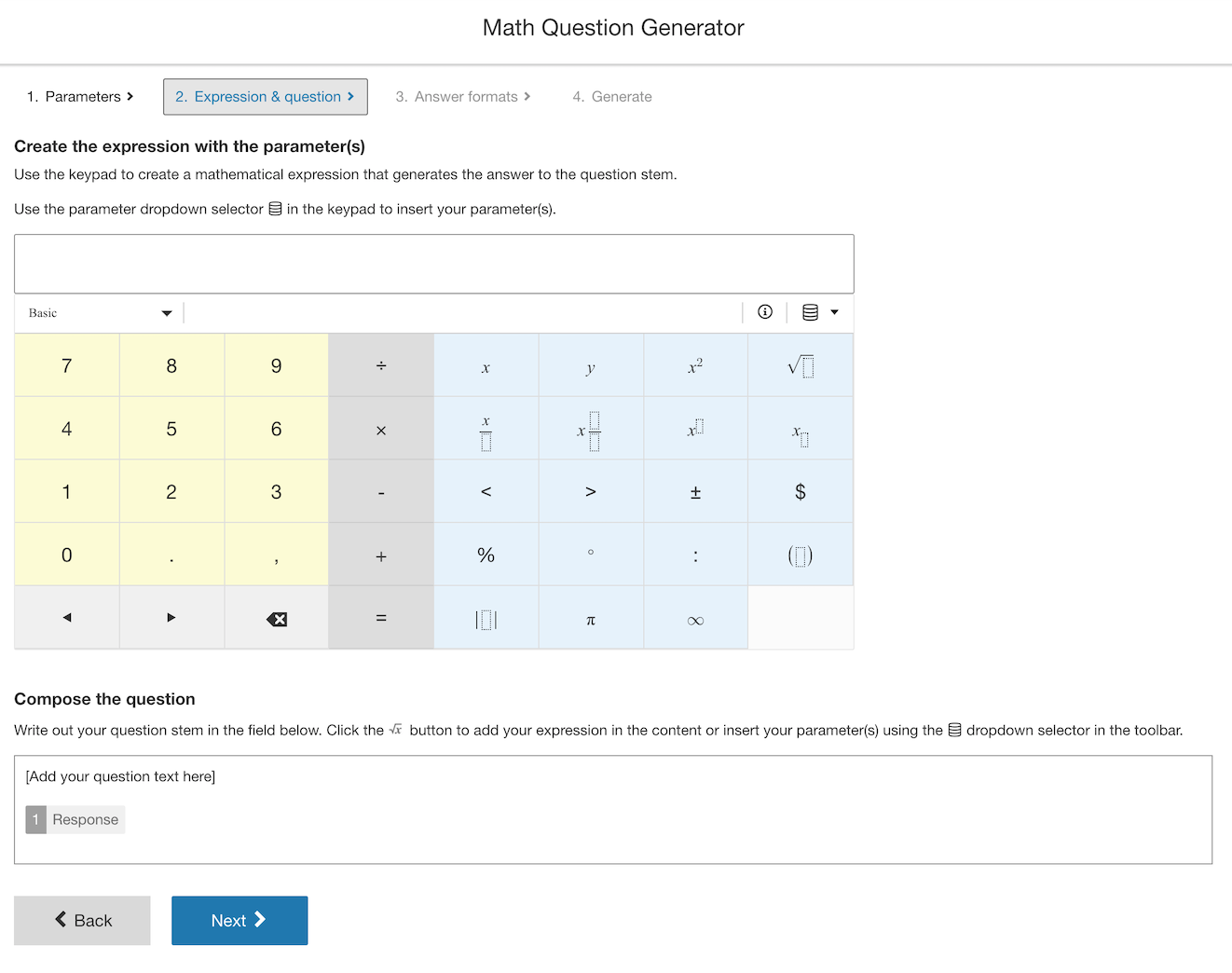Screenshot 3: creating your mathematical expression

In this section, the expression that defines the question is set including the parameters created previously. This expression is the basis for the validation setting (the correct answer) that is returned by Math question generator. It can also be used within the question content itself. See the headings 'Adding Parameters within an expression' and 'Adding Parameters inline with text' on this page for examples.

Using the available keypad, enter the required math, adding the parameters. These are available from the ‘Data'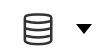dropdown.

There are two distinct ways that content created in math question generator can be used within an Item. The parameters can be applied directly within an expression, or they can be added inline within the question text.

### Adding parameters within an expression

Let’s take the example of factorizing a quadratic expression to demonstrate this use case.

`(x+a)(bx-c)`

Our constants are a, b and c and will become the parameters we set for generation of math question generator content.

As per Create parameters and set their values enter these parameters with the following values:
a - set a range 1 to 5
b - set a list containing 2,4,6
c - set a list containing 1,3,5

Now move to the Expression & question section and using the keypad add a mathematical expression that will form the basis of your question. This expression will be evaluated when generating the correct answer forms. The parameters can be inserted within the expression using the Datadropdown.

In this example it will be the factored form of the expression, it should look like this: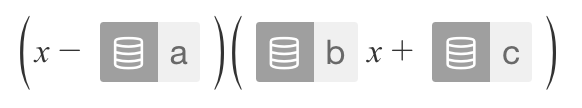Screenshot 4: an expression in the factored form.

Moving to the field below the keypad, this is where the question stem is added. This offers the same rich text options of a regular math question stimulus. Additionally, in math question generator, there is the 'use expression' option to place a copy of the expression into the question stem if required.

Below is how this example may be authored.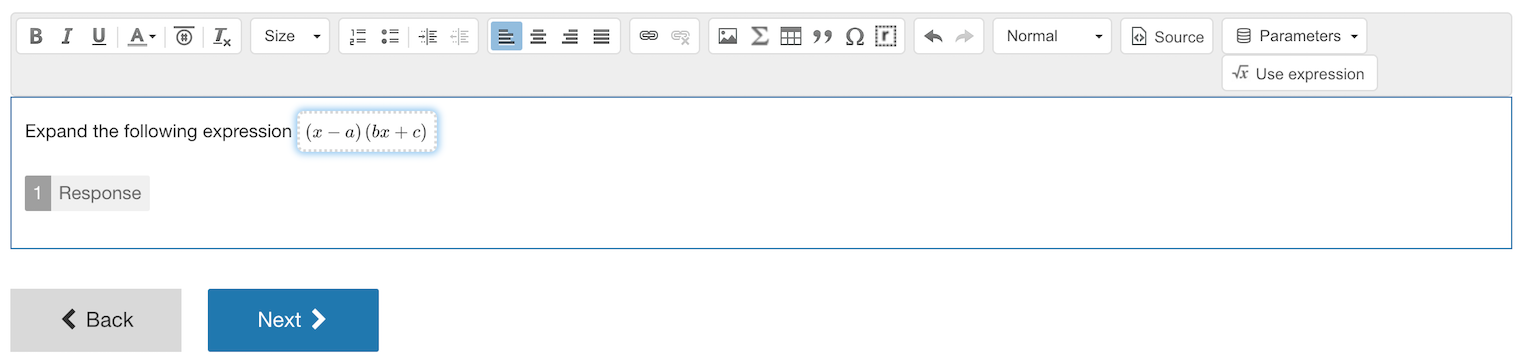Screenshot 5: Authoring your question stem.

Note: you must ensure that there is a ‘Response’ box included in the question text. This is the field that the user will enter their answer in, and where the Learnosity math engine will process the answer from. You can only add one ‘response’ area as we do not handle multiple responses with math question generator content.

The default size is 25px square to accommodate its use inline within text. If an alternate size is required this can be adjusted under the Layout section once math question generator content is set and you are back in the question editor. Select preview to see the result as it will be rendered.

### Adding parameters inline in text

Math question generator parameters can also be used within text to add the parameter values to the question stem itself.

Let's take the example of numbers of fruit. We will ask the user to calculate the final number of fruit a group of people have combined.

First, we need to define the fruit. On the first screen of the wizard we enter all the fruit parameters, we add ‘apples’, ‘bananas’ and ‘pears’ and set each parameter range to the desired amount. In this case, we set ranges of 1 to 5.

On the next screen we can define our expression and our question, the order does not matter. For this example we will set the question first, remember the parameters are available in the ‘data dropdown' buttonwithin the text editor toolbar. Don’t forget you need to have one response box in this section for the user to enter their answer.

See below: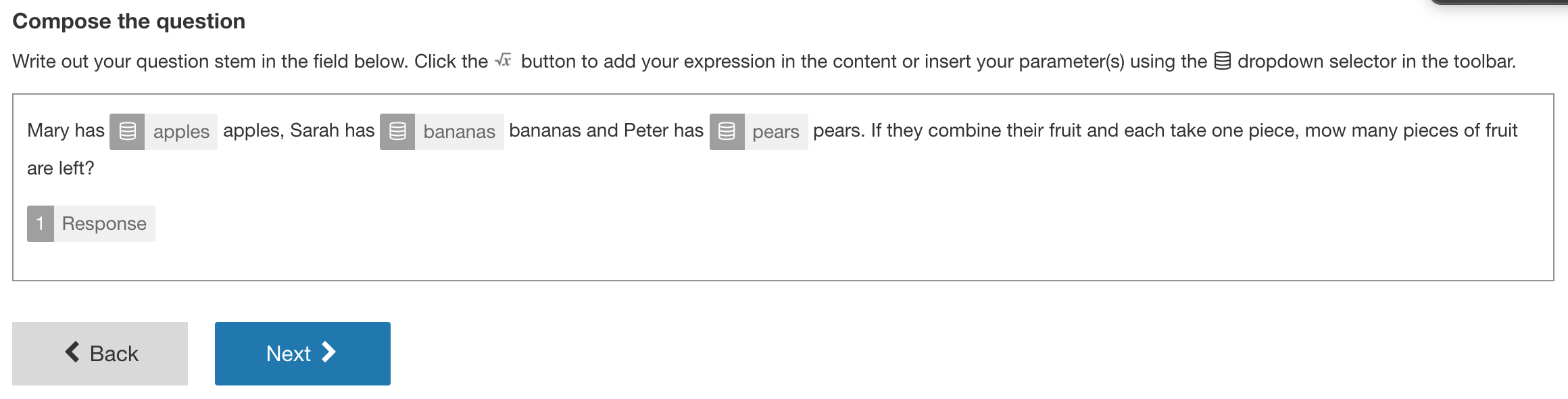Next, we will need to define an expression that represents this question. In the same way as the factored example, this expression will be used by math question generator  to calculate the correct answer in all adaptations.

In this case we need to add apples, bananas and pears and then subtract three.

So, we would enter the following: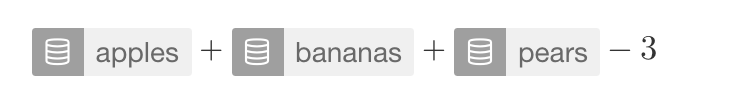## 3. Select the correct answer format(s)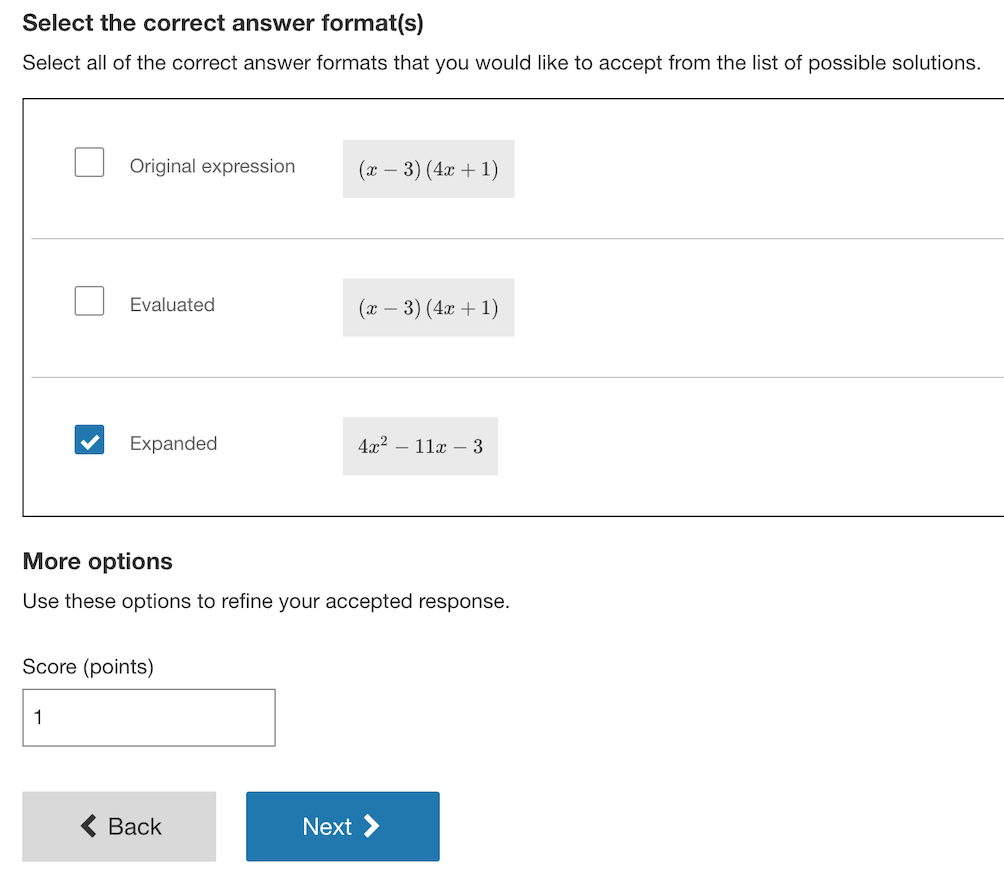Screenshot 8: Selecting the answer format.

Moving to the next section of the wizard, math question generator will process the expression and parameter settings and present you with all mathematical forms that represent a possible answer the Learnosity Math engine will accept. It will also offer any further settings that may be relevant to the form of the correct response. For example, if there are decimals or thousandths in the valid answers, configuration options are displayed to set the accepted forms of the decimal and thousandths separators.

The options displayed will be forms of a correct response that are relevant to the original expression set in 'Setting the question expression and creating the question stem'. Using the check boxes, select the forms of the correct answer that will be accepted.

NOTE: In some cases, only the original expression will be available to select. In this case it's recommended to check the original expression and the expected answer. Where this could be relevant for the intended use case, in most cases it signals that the original expression is invalid.

Behind the scenes, math question generator will automatically set the Learnosity math scoring method(s) for the question. Multiple forms of the solution can be selected and where relevant, alternate responses are set automatically, as well. Because setting the validation is handled automatically, the exiting math question validation interface is not displayed in the math question generator.

## 4. Generate the questions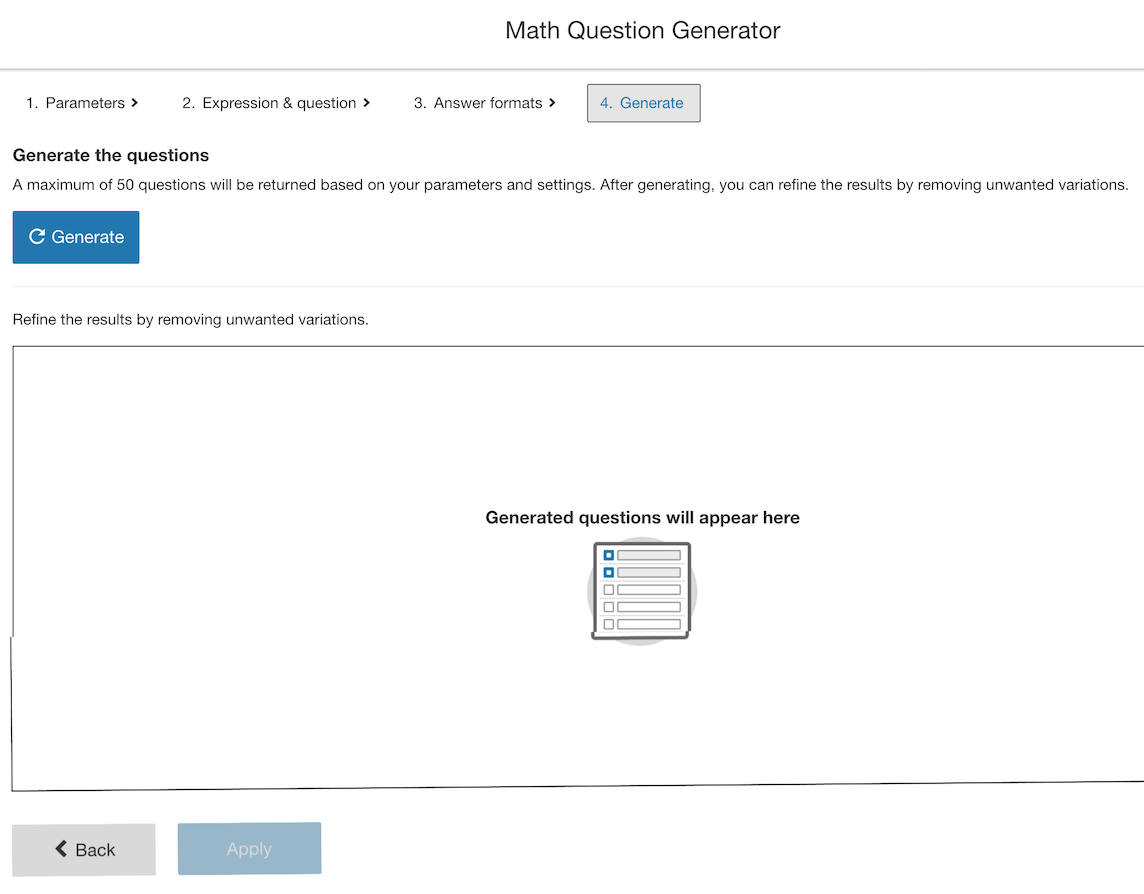The next section of the wizard will allow us to generate the possible variations for this expression. This is based on the ranges for each parameter, and the particular form of the correct answer(s).

Select 'generate' to display the populated table of possible variants of the equation.

Any unwanted variants can be removed from the final data table. As you scroll through the list of generated results, check those that you want to remove, then select the "Remove" button to remove these from the final data table saved to the item.

To ensure that the data table and the question stem content is committed to the item, it is necessary to select 'Apply'. The data and question content will be saved and returns to the question editor. Here, we can add further settings for the question and preview the results.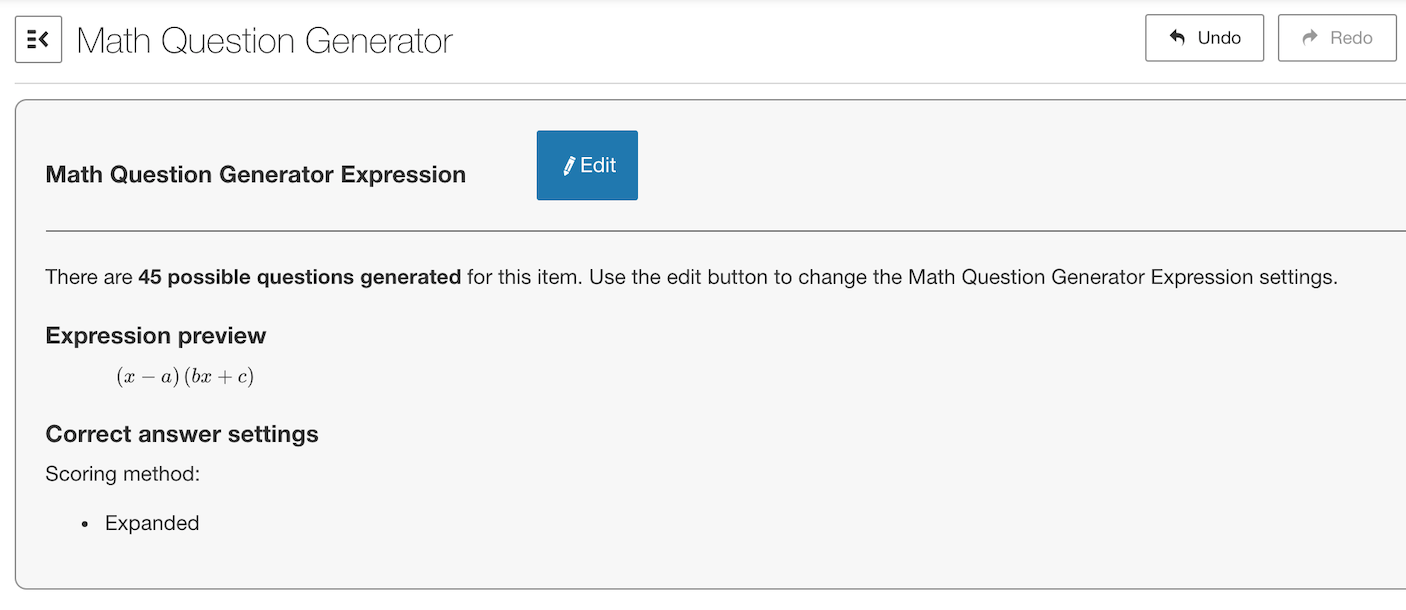Screenshot 10: The final view of your expression.